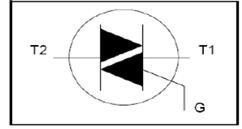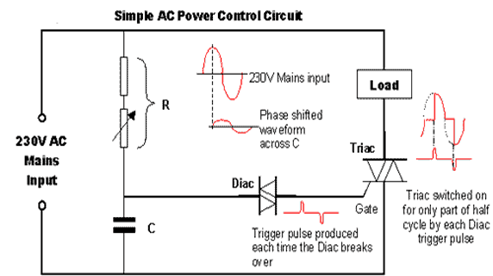# AC VOLTAGE CONTROL BY USING DIAC AND TRIAC

A TRIAC is a three terminal semiconductor device which can control the flow of the current and thus its name is TRIAC it has a similar operation to two thyristors connected in reverse parallel with a common gate connection. Thus it has the ability to be triggered into conductionTRIACS are used in power control to give full wave control and it could control the voltage between zero and full power Many industries face problems like voltage sags and extended under voltage and thus it could cause a negative impact on productivity for this we need to install voltage controllers to control the voltage TRIAC provides a wide range of control in AC circuit without the need for external components• We need to connect the load as lamp • We can see the variation of light by varying the variable resistor • There will be voltage and current reading at different steps of the load lamp or induction load would be there optionally • In CRO we can see the wave form connect the CRO and divide it by 10 and check the gate of TRIAC • Variation of phase angle could be seen while varying the potentiometer

The AC voltage controllers are of two types it is classified according to the type of input supply applied to the circuit • Single phase AC controllers • Three phase AC controllers

Single phase controllers operate with single phase supply voltage 230v at 50Hz In the case of three phase, it will be 400v at 50hz DIAC will have a break over voltage at the range of 30 volts and if a voltage less than this is applied to the polarity it would remain in high resistance with a flow of small leakage current

Application of AC voltage controller • Illumination control in AC circuits • Industrial heating, induction heating, and domestic heating • Tap changing of a transformer • Induction motor speed control • Control of AC magnets